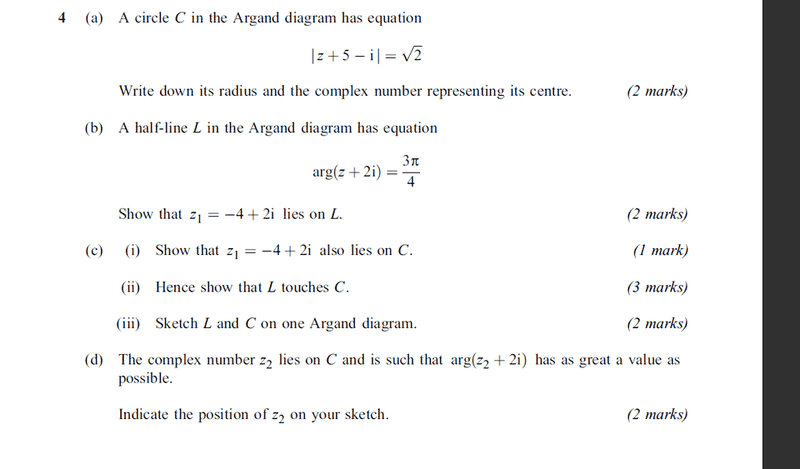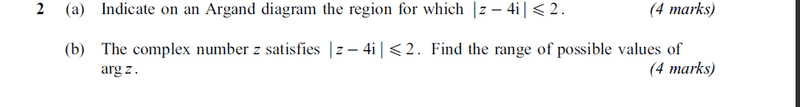# Argand Loci

ibysaiyan

## Homework Statement

Hi,
Can someone please explain me on how to do both of the question posted.
Honestly speaking as i type my head is aching real bad, having a total mental block.

## Homework Equations## The Attempt at a Solution

for question 2) i dont know which region to shade for all i know is that the radius is equal to 2 or less.As far as the finding out the ranges go arghh i am totally lost.All i remember is that arg(z-z) = constant. :(
For question 4 i managed to do part a but not the rest.
I might rest for awhile or something.I dont know :(.

## Answers and Replies

latentcorpse
for the first one consider this:

$|z-a|=r$ is the set of all points $\{ z \}$ a distance r from a i.e. it's a circle of radius r and centre a.
so for your example r is easy enough to obtain its just root 2. then just write the modulus bit in the same form as above to get a.

the next bit subsititute z1 in and find the arg of -4+4i recall $\tan{ arg(z) } = \frac{y}{x}$ for z=x+iy

so on so forth with the next one. just check when you put it into the LHS of circle equation you get the RHS to be root 2

part (iii) is a bit harder. i'd write the equation of the circle in the form x^2+y^2=r^2 and differentiate to get the equation of the tangent at teh point z1. now if you write the equation of L in the form y-b=m(x-a), they should hopefully match up. by showing L is tangent to C, you show they touch at that point and don't intersect.

next bit just sketch them. prettty easy.